.

# Anomaly Detection from Head and Abdominal Fetal ECG — A Case study of IOT anomaly detection using Generative Adversarial Networks

## Anomaly Detection from Head and Abdominal Fetal ECG — A Case study of IOT anomaly detection using Generative Adversarial Networks## Motivation

In this blog, we discuss the role of Variation Auto Encoder in detecting anomalies from fetal ECG signals.

Variational Auto Encoder ways to accurately determine anomalies from seasonal metrics occurring at regular intervals ( i.e. daily/weekly/bi-weekly/monthly or periodic events at finer granular levels of mins/secs) so as to facilitate timely actions from the concerned team. Such timely actions help to recover from serious issues such as predictive maintenance) in the field of web applications, retail, IoT, telecom, and healthcare industry.

The metrics/KPIs that plays an important role in determining anomalies are composed of noises that are assumed to be independent, zero-mean Gaussian at every point. In fact, the seasonal KPIs comprises of seasonal patterns with local variations, and statistics of the Gaussian noises.

## Role of IoT/Wearables

Portable low-power fetal ECG collectors like wearables have been designed for research and analysis and, which can collect maternal abdominal ECG signals in real-time. The ECG data can be sent to a smartphone client via Bluetooth to individually analyze signals captured from the fetal brain and maternal abdomen. The extracted fetal ECG signals can be used to detect any anomaly in fetal behavior.

## Variation Auto-Encoder

Deep Bayesian networks employ black-box learning patterns with neural networks to express the relationships between variables in the training dataset. Variational Auto Encoders are nothing but Deep Bayesian Networks which are often used in training and prediction, uses Neural Networks to model posteriors of the distributions.

Variational Auto Encoders (VAEs) supports optimization by setting a lower bound on the likelihood via a reparameterization of the Evidence Lower Bound (ELBO). The ELBO method uses a 2 step process of maximizing the log-likelihood, the likelihood tries to make the generated sample (image/data) more correlated to the latent variable, which makes the model more deterministic. In addition, it minimizes the KL divergence between the posterior and the prior.

## Characteristics/Architecture of DoNut

The Donut recognizes the normal pattern of a partially abnormal x, and find a good posterior in order to estimate how well x follows the normal pattern. The fundamental characteristic of Donut is to enhance its ability to find good posteriors by reconstructing normal points within abnormal windows. This property is infused in its training property by M-ELBO (Modified ELBOW), that turns out to be superior, in contrast to excluding all windows containing anomalies and missing points from the training data.

Thus summarizing the three techniques employed in VAE based anomaly detection algorithm in Donut architecture includes the following:

• Modified ELBO – Ensures that an average, a certain minimum number of bits of information are encoded per latent variable, or per group of the latent variable. This helps to increase the information capacity and reconstruction accuracy.
• Missing Data Injection for training – A kind of data augmentation procedure used to fill the missing points as zeros. It amplifies the effect of ELBO by injecting the missing data before the training epoch starts and recovering the missing points after the epoch is finished.
• MCMC Imputation for better anomaly detection – Improves posterior estimation by synthetically generated missing points.The network structure of Donut. Gray nodes are random variables, and white nodes are layers.

The data preparation stage deals with Standardization, Missing value Injection and grouping data in terms of Sliding Window (length say (W) over key metrics), where each point xt is being processed as xt−W +1, . . . , x. The training process encompasses Modified ELBO and Missing Data Injection. In the final prediction stage, MCMC Imputation (as shown in the figure below) is applied to yield a better posterior distribution.MCMC Imputation and Anomaly Detection

Source (Unsupervised Anomaly Detection via Variational Auto-Encoder for Seasonal KPIs in Web Applications

To know more about ELBO in VATE check out https://medium.com/@hfdtsinghua/derivation-of-elbo-in-vae-25ad7991fdf7 or refer to the references below.

File Imports

``import numpy as np from donut import complete_timestamp, standardize_kpi import pandas as pd import csv import matplotlib.pyplot as plt import seaborn as sns sns.set(rc={'figure.figsize':(11, 4)}) from sklearn.metrics import accuracy_score import mne import pandas as pd import numpy as np import matplotlib.pyplot as plt``

Here we add timestamps to the Fetal ECG data, under the assumption that each data point is recorded at an interval of 1 second, (although the data-set source suggests that the signal are recorded at 1 Khz.). We further resample the data at an interval of 1 minute by taking an average of 60 samples.

``data_path = '../abdominal-and-direct-fetal-ecg-database-1.0.0/' file_name = 'r10.edf'  edf = mne.io.read_raw_edf(data_path+file_name) header = ','.join(edf.ch_names) np.savetxt('r10.csv', edf.get_data().T, delimiter=',', header=header)  df = pd.read_csv('r10.csv') periods = df.shape  dti = pd.date_range('2018-01-01', periods=periods, freq='s') print(dti.shape, df.shape) df['DateTs'] = dti  df.set_index('DateTs') df.index = pd.to_datetime(df.index, unit='s') df1 = df.resample('1T').mean()``

Once the data is indexed by time-stamps we plot the individual features and try to explore seasonality patterns if any. We also add a label feature metric, signifying potential anomalies that could be present in the input data by considering at high-level of brain signal fluctuations (>= .00025 and <= -.00025). We chose the brain signal, as it closely resembles the signal curves and spikes of 4 other abdominal signals.

### Data Labelling and Plotting the Features

As there are total 5 signals (one from fetal brain and 4 from abdomen

``df1.rename_axis('timestamp', inplace=True) print(cols, df1.index.name)  df1['label'] =  np.where((df1['# Direct_1'] >= .00025) | (df1['# Direct_1'] <= -.00025), 1, 0) print(df1.head(5))  for i in range(0, len(cols)):     if(cols[i] != 'timestamp'):         plt.figure(figsize=(20, 10))         plt.plot(df1[cols[i]], marker='^', color='red')         plt.title(cols[i])         plt.savefig('figs/f_' + str(i) + '.png')``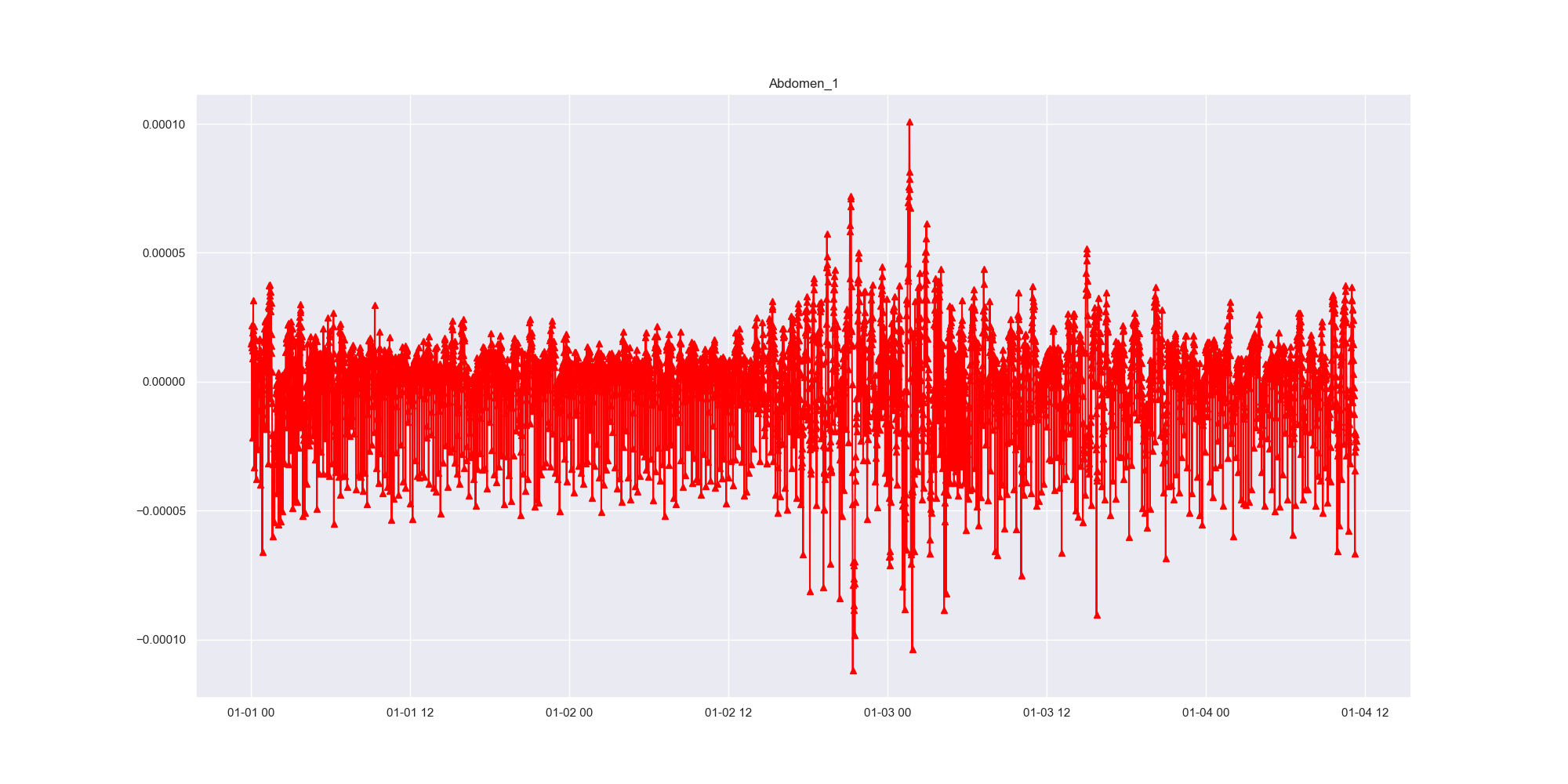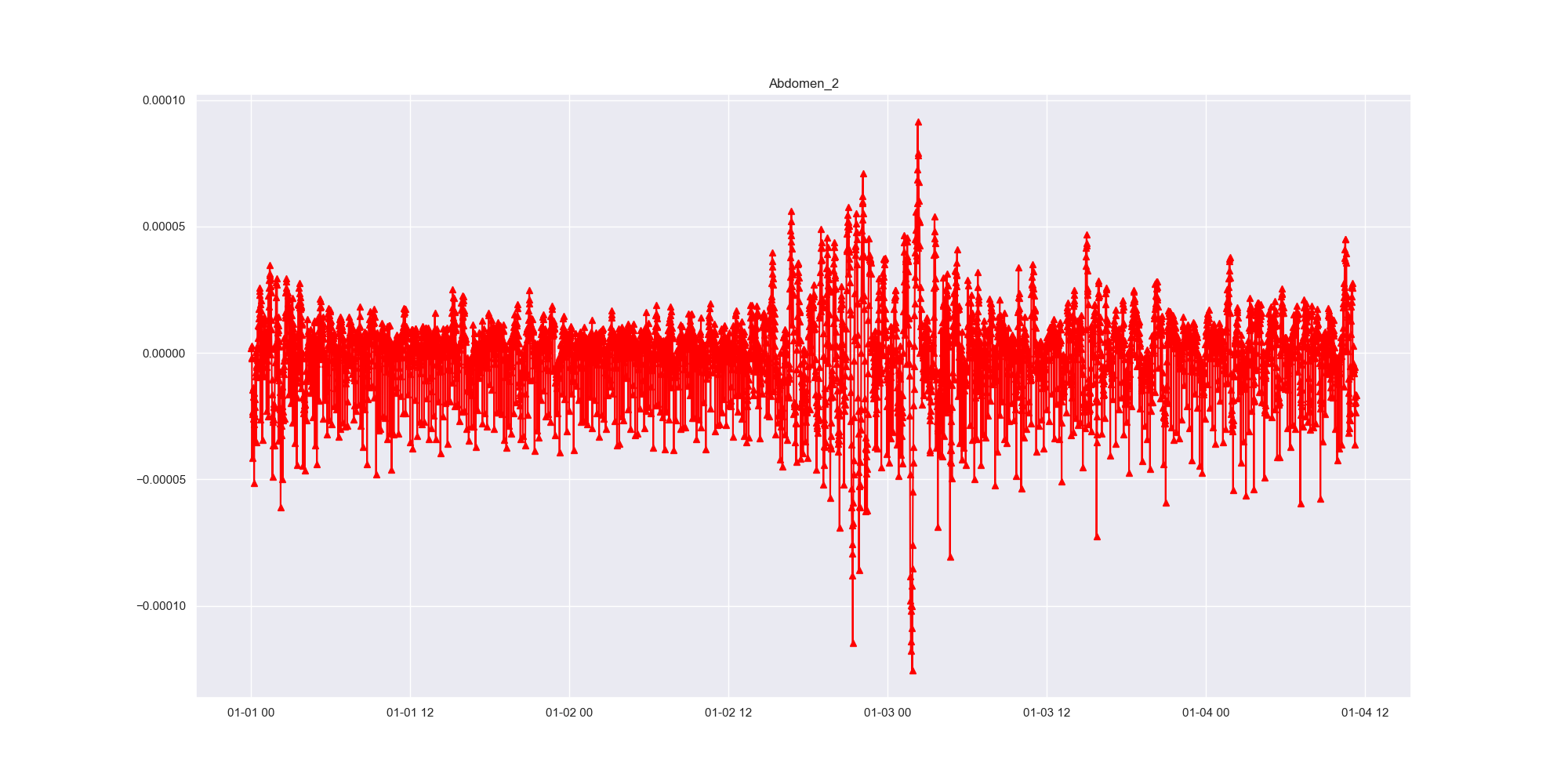### Training the data using Adversarial Networks

``df2 = df1.reset_index() df2 = df2.reset_index(drop=True) #drop the index, instead use as it as a feature vector before discovering the missing data points  # Read the raw data for 1st feature Direct_1 timestamp, values, labels = df2['timestamp'], df2['# Direct_1'], df2['label'] # If there is no label, simply use all zeros. labels = np.zeros_like(values, dtype=np.int32)   # Complete the timestamp, and obtain the missing point indicators. timestamp, missing, (values, labels) = \     complete_timestamp(timestamp, (values, labels))   # Split the training and testing data. test_portion = 0.3 test_n = int(len(values) * test_portion) train_values, test_values = values[:-test_n], values[-test_n:] train_labels, test_labels = labels[:-test_n], labels[-test_n:] train_missing, test_missing = missing[:-test_n], missing[-test_n:]  # Standardize the training and testing data. train_values, mean, std = standardize_kpi(     train_values, excludes=np.logical_or(train_labels, train_missing)) test_values, _, _ = standardize_kpi(test_values, mean=mean, std=std)   import tensorflow as tf from donut import Donut from tensorflow import keras as K from tfsnippet.modules import Sequential from donut import DonutTrainer, DonutPredictor   # We build the entire model within the scope of `model_vs`, # it should hold exactly all the variables of `model`, including # the variables created by Keras layers. with tf.variable_scope('model') as model_vs:     model = Donut(         h_for_p_x=Sequential([             K.layers.Dense(50, kernel_regularizer=K.regularizers.l2(0.001),                            activation=tf.nn.relu),             K.layers.Dense(50, kernel_regularizer=K.regularizers.l2(0.001),                            activation=tf.nn.relu),         ]),         h_for_q_z=Sequential([             K.layers.Dense(50, kernel_regularizer=K.regularizers.l2(0.001),                            activation=tf.nn.relu),             K.layers.Dense(50, kernel_regularizer=K.regularizers.l2(0.001),                            activation=tf.nn.relu),         ]),         x_dims=120,         z_dims=5,     )   trainer = DonutTrainer(model=model, model_vs=model_vs, max_epoch=512) predictor = DonutPredictor(model)   with tf.Session().as_default():     trainer.fit(train_values, train_labels, train_missing, mean, std)     test_score = predictor.get_score(test_values, test_missing)      pred_score = np.array(test_score).reshape(-1, 1)     print(len(test_missing), len(train_missing), len(pred_score), len(test_values))     y_pred = np.argmax(pred_score, axis=1)``

The model is trained with default parameters as listed below:

`use_regularization_loss=True, max_epoch=512,  batch_size=256, valid_batch_size=1024,  valid_step_freq=100, initial_lr=0.001,  optimizer=tf.train.AdamOptimizer,  grad_clip_norm=10.0 #Clip gradient by this norm. `

The model summary with its trainable parameters, number of hidden layers can be obtained as :

`Trainable Parameters (24,200 in total) donut/p_x_given_z/x_mean/bias (120,) 120 donut/p_x_given_z/x_mean/kernel (50, 120) 6,000 donut/p_x_given_z/x_std/bias (120,) 120 donut/p_x_given_z/x_std/kernel (50, 120) 6,000 donut/q_z_given_x/z_mean/bias (5,) 5 donut/q_z_given_x/z_mean/kernel (50, 5) 250 donut/q_z_given_x/z_std/bias (5,) 5 donut/q_z_given_x/z_std/kernel (50, 5) 250 sequential/forward/_0/dense/bias (50,) 50 sequential/forward/_0/dense/kernel (5, 50) 250 sequential/forward/_1/dense_1/bias (50,) 50 sequential/forward/_1/dense_1/kernel (50, 50) 2,500 sequential_1/forward/_0/dense_2/bias (50,) 50 sequential_1/forward/_0/dense_2/kernel (120, 50) 6,000 sequential_1/forward/_1/dense_3/bias (50,) 50 sequential_1/forward/_1/dense_3/kernel (50, 50) 2,500 `
`This model is obtained from the following code snippet:  model = Donut( h_for_p_x=Sequential([ K.layers.Dense(50, kernel_regularizer=K.regularizers.l2(0.001), activation=tf.nn.relu), K.layers.Dense(50, kernel_regularizer=K.regularizers.l2(0.001), activation=tf.nn.relu), ]), h_for_q_z=Sequential([ K.layers.Dense(50, kernel_regularizer=K.regularizers.l2(0.001), activation=tf.nn.relu), K.layers.Dense(50, kernel_regularizer=K.regularizers.l2(0.001), activation=tf.nn.relu), ]), x_dims=120, z_dims=5, )`

This DoNut Network contains uses The variational auto-encoder (“Auto-Encoding Variational Bayes”,Kingma, D.P. and Welling) which is a deep Bayesian network, with observed variable x and latent variable z. The VAE is generated using TFSnippet (library for writing and testing tensorflow models). The generative process of Auto-Encoder is initiated with parameter z with prior distribution p(z), and a hidden network h(z), then uses observed variable x with distribution p(x | h(z)). The posterior inference p(z | x), variational inference techniques are adopted, to train a separated distribution q(z | h(x)).

Here each Sequential function creates a multi-layer perception, with 2 hidden layers of 50 units and RELU activation. The 2 distributions “h_for_p_x” and “h_for_q_z“, are created using the same Sequential function (as evident from Model Summary (Sequential and Sequential_1) and they represent the hidden networks for “p_x_given_z” and “q_z_given_x”.

## Plotting the Anomalies/Non-Anomalies together or Individually

We plot the anomalies (in red) together with non-anomalies (green) and also try to superimpose both of them together in the same graph so as to analyze the combined impact.

In the Donut prediction, the higher the prediction score the data is less anomalous. We prefer to choose (-3) as the threshold margin of predicting anomalous points.

We also compute the number of inliers and outliers and plot them against time-stamped values along the x-axis.

``    plt.figure(figsize=(20, 10))     split_test  = int((test_portion)*df.shape)      anomaly = np.where(pred_score > -3, 0, 1)      df3 = df2.iloc[-anomaly.shape:]     df3['outlier'] = anomaly     df3.reset_index(drop=True)      print(df3.head(2), df3.shape)     print("Split", split_test, df3.shape)     di = df3[df3['outlier'] == 0]     do = df3[df3['outlier'] == 1]      di = di.set_index(['timestamp'])     do = do.set_index(['timestamp'])      print("Outlier and Inlier Numbers", do.shape, di.shape, di.columns, do.columns)      outliers = pd.Series(do['# Direct_1'], do.index)     inliers = pd.Series(di['# Direct_1'], di.index)      plt.plot(do['# Direct_1'], marker='^', color='red', label="Anomalies")     plt.plot(di['# Direct_1'],  marker='^', color='green', label="Non Anomalies")      plt.legend(['Anomalies', 'Non Anomalies'])     plt.title('Anomalies and Non Anomalies from Fetal Head Scan')     plt.show()      di = di.reset_index()     do = do.reset_index()     plt.figure(figsize=(20, 10))      do.plot.scatter(y ='# Direct_1', x = 'timestamp', marker='^', color='red', label="Anomalies")      plt.legend(['Anomalies'])     plt.xlim(df3['timestamp'].min(), df3['timestamp'].max())     plt.ylim(-.0006, .0006)     plt.title('Anomalies from Fetal Head Scan')     plt.show()     plt.figure(figsize=(20, 10))     di.plot.scatter(y='# Direct_1', x='timestamp', marker='^', color='green', label="Non Anomalies")     plt.legend(['Non Anomalies'])     plt.xlim(df3['timestamp'].min(), df3['timestamp'].max())     plt.ylim(-.0006, .0006)     plt.title('Non Anomalies from Fetal Head Scan')     plt.show()``

## Anomaly Plots for Direct electrocardiogram recorded from fetal head

The three consecutive plot displays anomalous and non-anomalous points plotted against each other or separately as labeled, especially for signals obtained from Fetal Head Scan.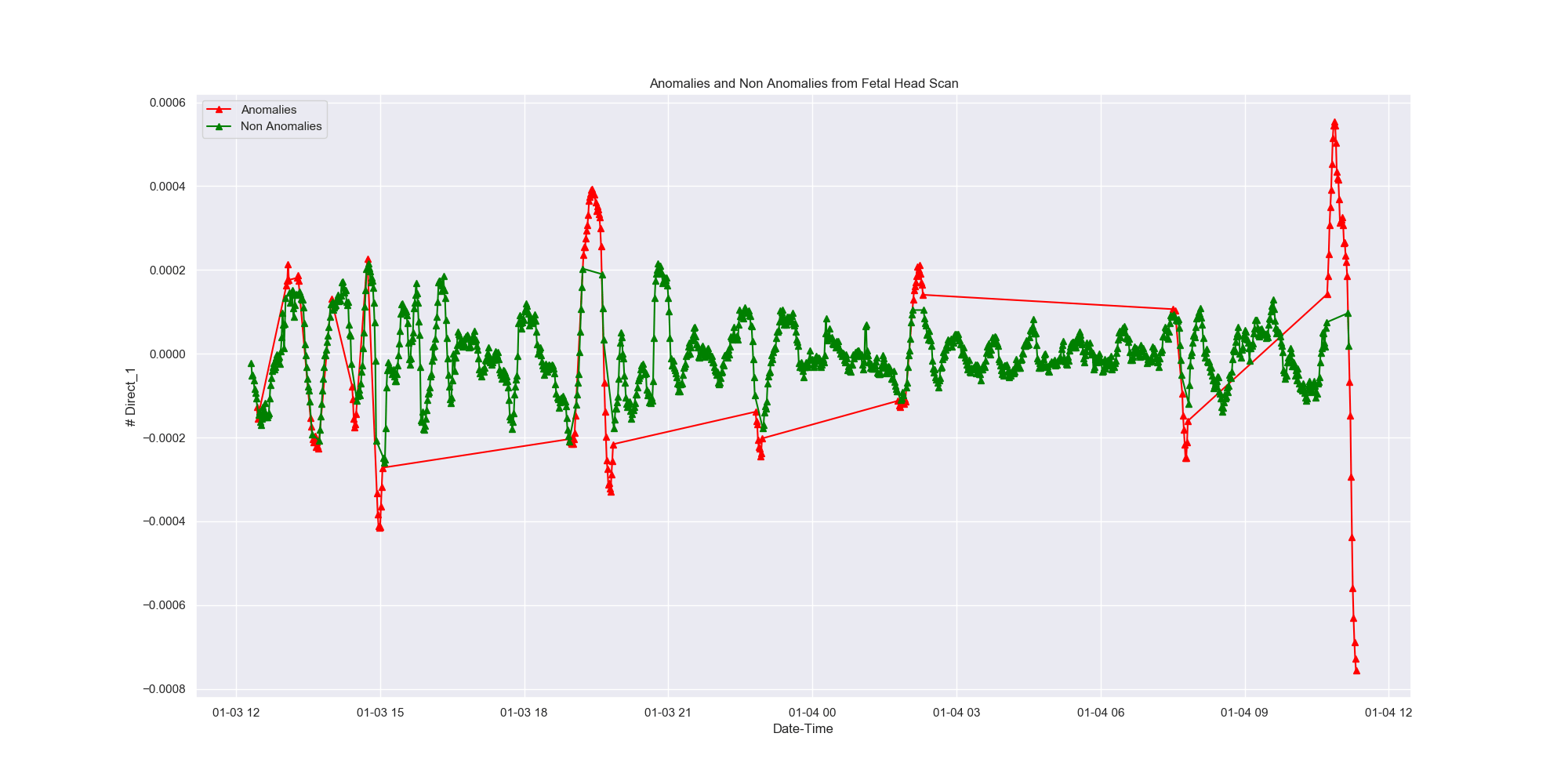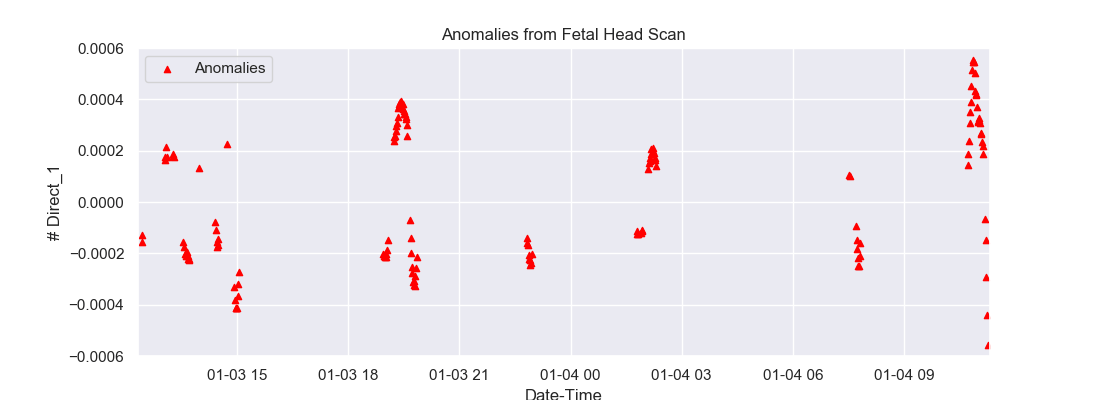## Anomaly Plots for Direct electrocardiogram recorded from maternal abdomen

The three consecutive plot displays anomalous and non-anomalous points plotted against each other or separately as labeled, especially for signals obtained from Fetus’s Maternal Abdomen.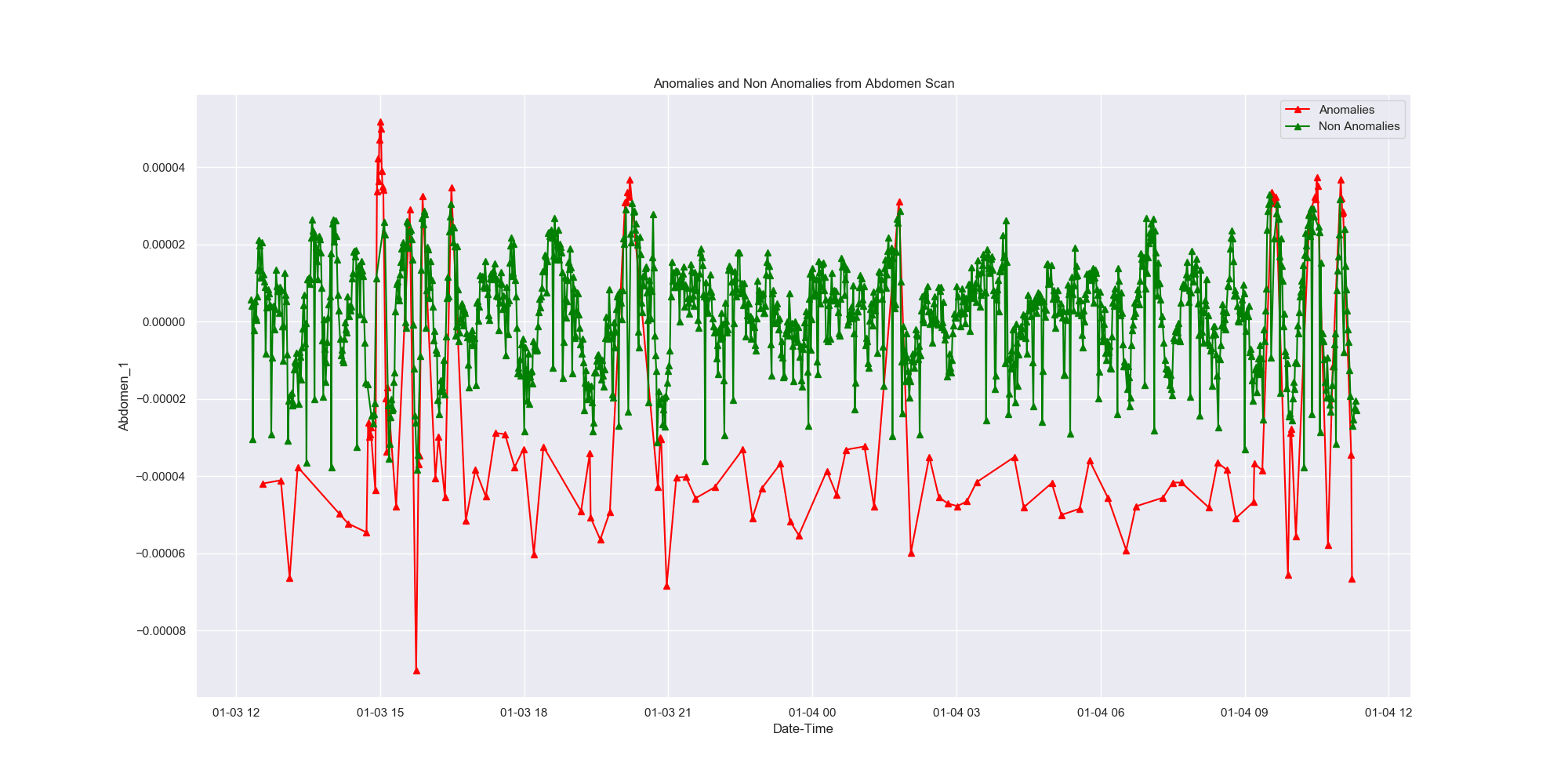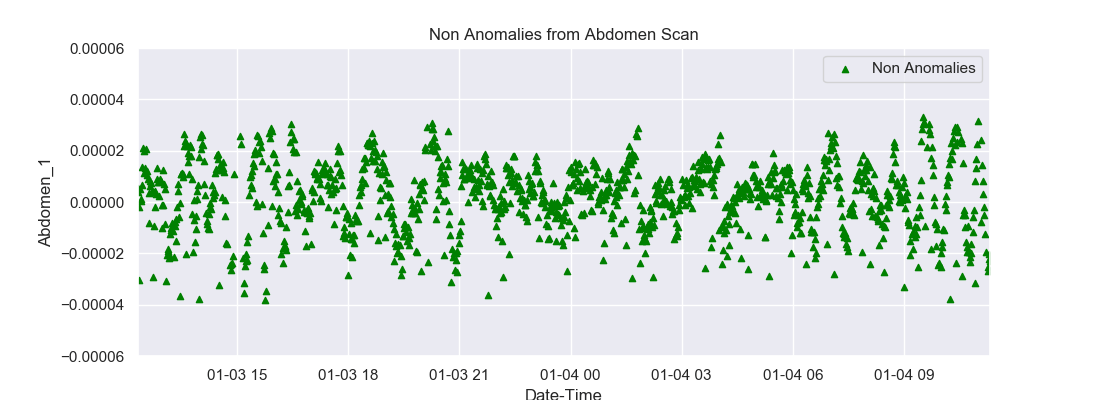=

## Conclusion

Some of the key. learnings of the Donut Architecture are:

• Dimensionality reduction based anomaly detection techniques need to use reconstruction mechanism to identify the variance and consequently identify the anomalies.
• Anomaly detection with generative models needs to train with both normal and abnormal data.
• Not relying on data imputation by any algorithm weaker than VAE, as this may degrade the performance.
• In order to discover the anomalies fast, the reconstruction probability for the last point in every window of x is computed.

We should also explore other variants of Auto Encoders (RNN, LSTM, LSTM with Attention Networks, Stacked Convolutional Bidirectional LSTM) in discovering anomalies for IoT devices.

The complete source code is available at https://github.com/sharmi1206/featal-ecg-anomaly-detection